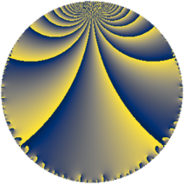# Properties

 Label 48.4.jLevel $48$ Weight $4$ Character orbit 48.j Rep. character $\chi_{48}(13,\cdot)$ Character field $\Q(\zeta_{4})$ Dimension $24$ Newform subspaces $1$ Sturm bound $32$ Trace bound $0$

# Related objects

## Defining parameters

 Level: $$N$$ $$=$$ $$48 = 2^{4} \cdot 3$$ Weight: $$k$$ $$=$$ $$4$$ Character orbit: $$[\chi]$$ $$=$$ 48.j (of order $$4$$ and degree $$2$$) Character conductor: $$\operatorname{cond}(\chi)$$ $$=$$ $$16$$ Character field: $$\Q(i)$$ Newform subspaces: $$1$$ Sturm bound: $$32$$ Trace bound: $$0$$

## Dimensions

The following table gives the dimensions of various subspaces of $$M_{4}(48, [\chi])$$.

Total New Old
Modular forms 52 24 28
Cusp forms 44 24 20
Eisenstein series 8 0 8

## Trace form

 $$24q - 20q^{4} + 84q^{8} + O(q^{10})$$ $$24q - 20q^{4} + 84q^{8} + 72q^{10} - 40q^{11} - 24q^{12} - 348q^{14} + 120q^{15} - 192q^{16} - 36q^{18} + 24q^{19} + 80q^{20} + 704q^{22} + 228q^{24} - 20q^{26} - 344q^{28} + 400q^{29} - 408q^{30} - 744q^{31} - 960q^{32} - 704q^{34} - 456q^{35} + 108q^{36} + 16q^{37} + 1256q^{38} + 1744q^{40} + 660q^{42} + 1240q^{43} - 200q^{44} - 1432q^{46} - 528q^{48} - 1176q^{49} + 708q^{50} + 744q^{51} + 1008q^{52} + 752q^{53} + 108q^{54} + 1344q^{56} + 1936q^{58} - 1376q^{59} - 1224q^{60} - 912q^{61} - 996q^{62} - 504q^{63} - 56q^{64} + 976q^{65} - 1368q^{66} - 2256q^{67} - 1568q^{68} - 528q^{69} - 1760q^{70} - 612q^{72} - 2740q^{74} + 1104q^{75} - 1880q^{76} + 1904q^{77} + 1692q^{78} + 5992q^{79} + 712q^{80} - 1944q^{81} - 40q^{82} + 2680q^{83} + 1800q^{84} - 240q^{85} - 1712q^{86} - 3936q^{88} + 648q^{90} - 3496q^{91} + 5296q^{92} + 5272q^{94} - 7728q^{95} + 2880q^{96} + 6760q^{98} - 360q^{99} + O(q^{100})$$

## Decomposition of $$S_{4}^{\mathrm{new}}(48, [\chi])$$ into newform subspaces

Label Dim. $$A$$ Field CM Traces $q$-expansion
$$a_2$$ $$a_3$$ $$a_5$$ $$a_7$$
48.4.j.a $$24$$ $$2.832$$ None $$0$$ $$0$$ $$0$$ $$0$$

## Decomposition of $$S_{4}^{\mathrm{old}}(48, [\chi])$$ into lower level spaces

$$S_{4}^{\mathrm{old}}(48, [\chi]) \cong$$ $$S_{4}^{\mathrm{new}}(16, [\chi])$$$$^{\oplus 2}$$